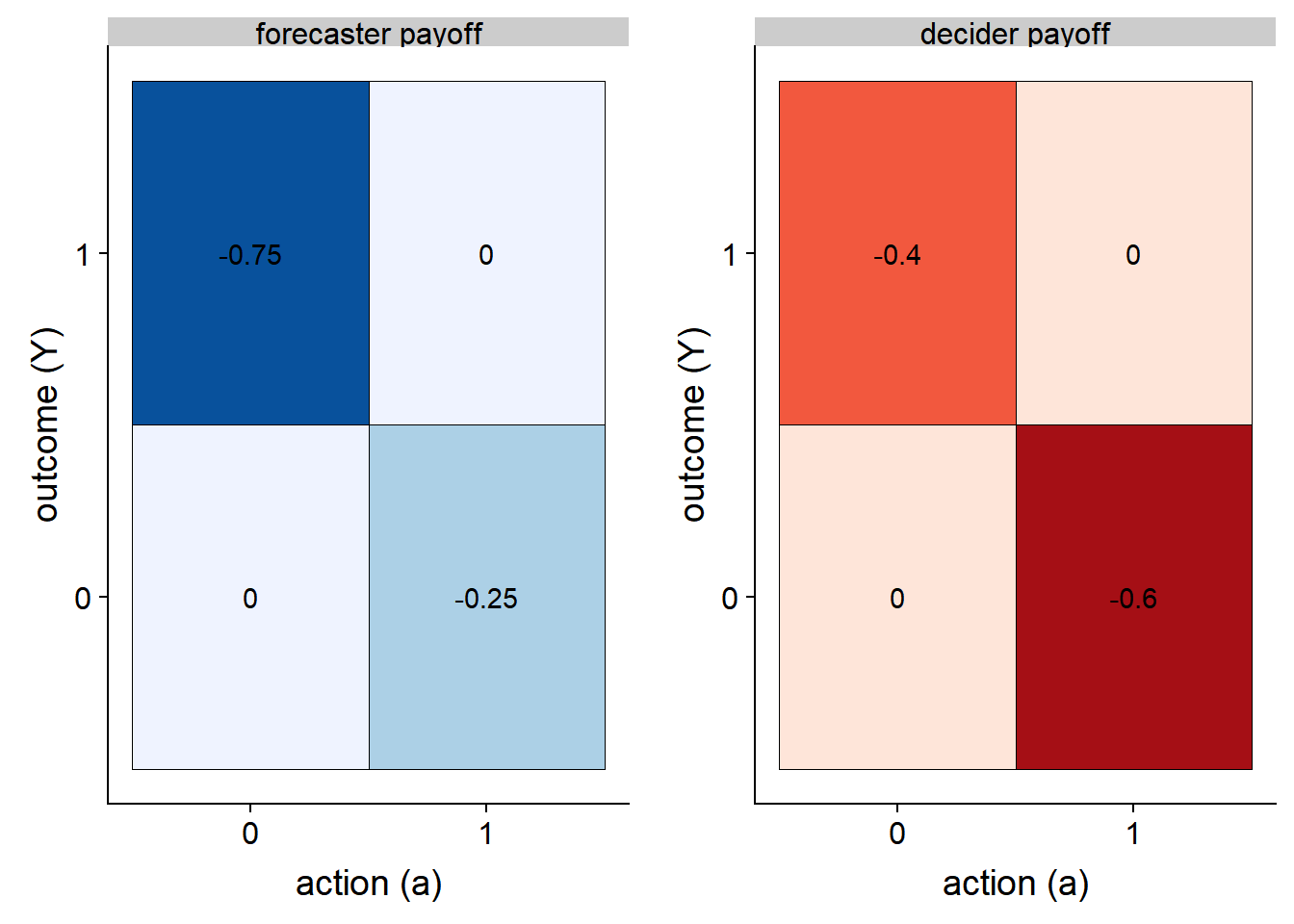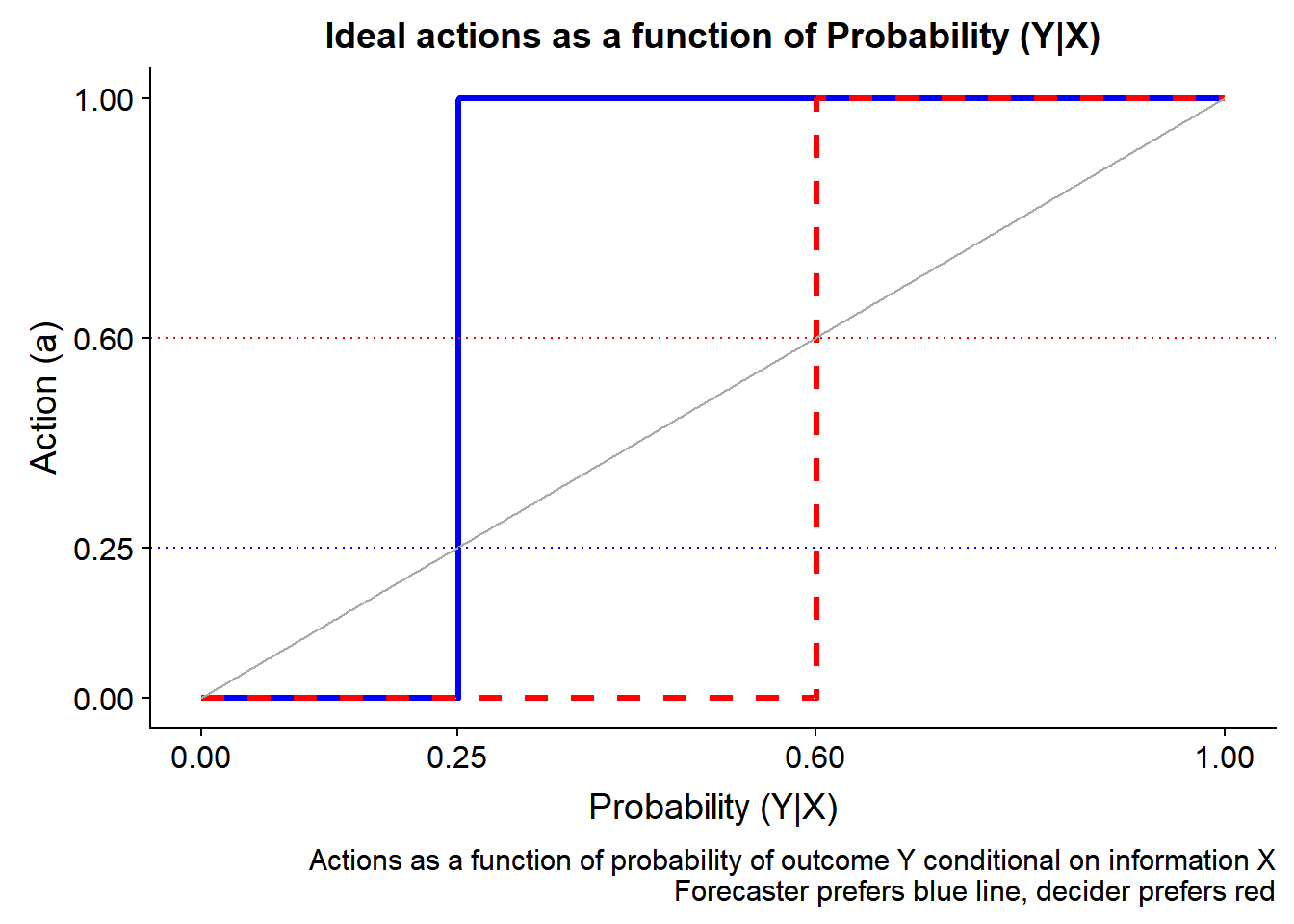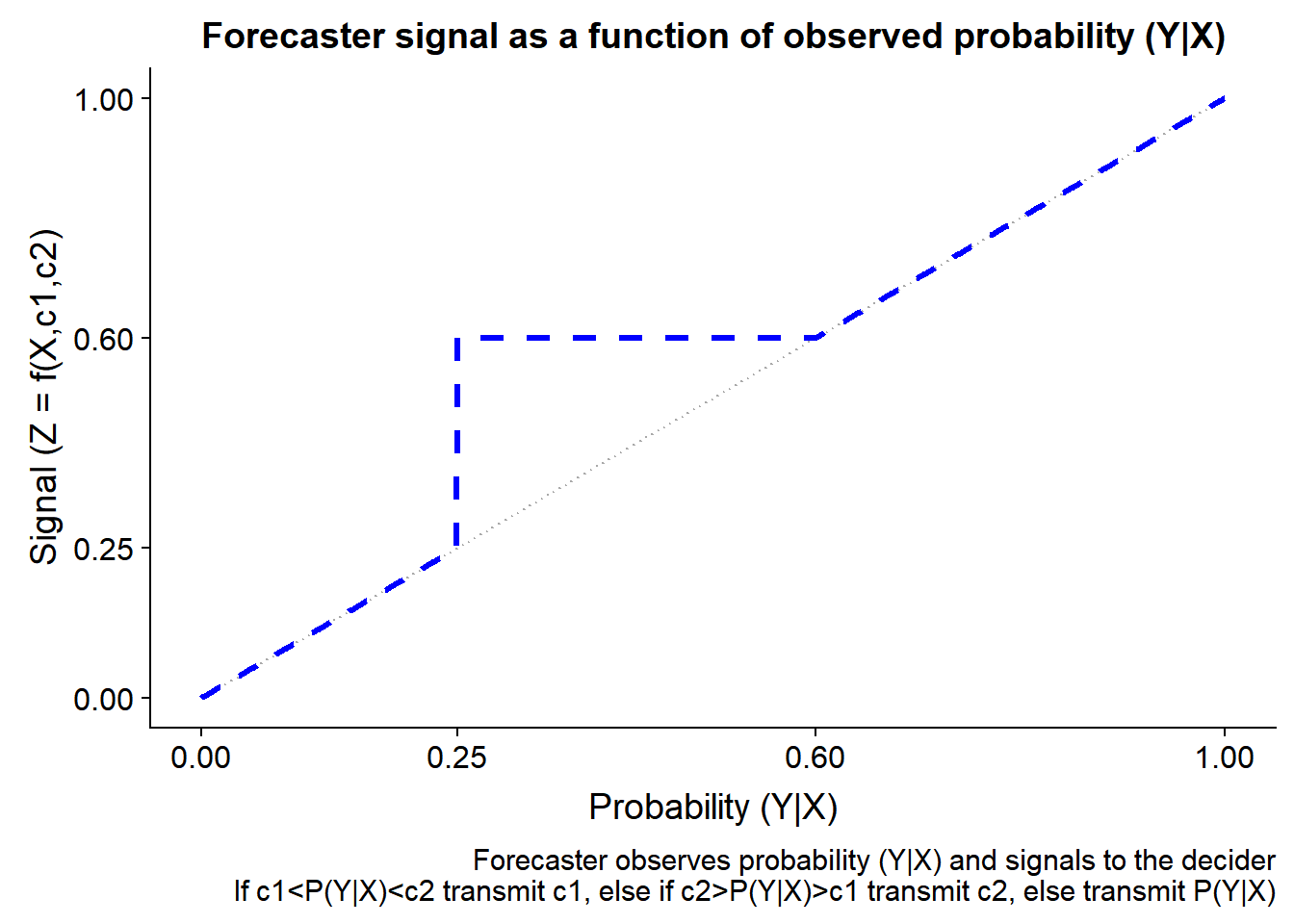# Forecasting and deciding binary outcomes under asymmetric information

## What's the best way for a forecaster to communicate when their payoff for a binary outcome differs from the decision maker? Guys, it's time for some game theory.

LAST WEEK IN THE WALL STREET JOURNAL an article LINK talked about how pundits can strategically make probabilistic forecasts. It seems 40% is a sort of magic number, where it’s high enough that if the event comes true you can claim credit as a forecaster, but if it doesn’t happen, you still gave it less than 50/50 odds.

Since I’m often asked to make forecasts I’m interested in this problem. Under what conditions is a 40 percent probability an optimal forecast? Guys, it’s time for some game theory.

# Some literature

Per usual, there’s a literature on this topic. I’m going to point out two papers that I’ll draw from here.

• Elliott, G., Ghanem, D., & Krüger, F. (2016). Forecasting conditional probabilities of binary outcomes under misspecification. Review of Economics and Statistics, 98(4), 742-755. LINK to pdf

• Elliott, G., & Lieli, R. P. (2013). Predicting binary outcomes. Journal of Econometrics, 174(1), 15-26. Link to Journal of Econometrics, Link to Working Paper

Those papers do much more complicated things that we’ll do here, but they provide the basic framework I’m going to describe here. They are also very good on their own so you might want to check them out.

## Setup

I’m considering the following stylized setup. There are two agents, a forecaster and a decision maker (decider). The forecaster has access to some information (technology, special domain knowledge, model) that allows them to produce superior estimates of the probability of a future binary (0/1) outcome. For example, the forecaster may know something about predicting recessions or some other outcome of consequence. The decider has no special information, but has the ability to take a binary (0/1) action in response to the information provided by the forecaster. Both the forecaster and the decider get a payoff from the outcome and the action.

Where things get interesting is that we might suppose that the decider and the forecaster have different payoff function. For example, the consequences of taking an action could differ in that the forecaster suffered more from taking no action when the outcome warranted it, while the decider suffered more by taking the wrong action.

A graph might help:

#####################################################################################
## Step 0: Load Libraries ##
#####################################################################################
library(tidyverse)
library(cowplot)

#####################################################################################
## Step 1: Set up Parameters ##
#####################################################################################

# X variable (between 0 1)
X<-seq(0,1,.001)
c1<-0.25    # low cutoff
c2<-0.60    # high cutoff

df<-data.frame(x=X,
y1=1*(X>c1),
y2=1*(X>c2),
z1=X*(X<min(c1,c2))+
max(c1,c2)*(X>=min(c1,c2) & X <= max(c1,c2))+
(X>max(c1,c2))*X,
z2=
X*(X<min(c1,c2))+
min(c1,c2)*(X>=min(c1,c2) & X <= max(c1,c2))+
(X>max(c1,c2))*X
) %>% mutate(z=(c1<c2)*z1+(c1>=c2)*z2)

# a payoff function:
PAYOFFS<- data.frame (a=c(1,1,0,0), y=c(1,0,1,0), forecaster=c(0,-c1,-(1-c1),0), decider=c(0,-c2,-(1-c2),0)) %>% gather(player,payoff, -a,-y)
g.pd<-
ggplot(data=filter(PAYOFFS,player=="decider"), aes(x=factor(a),y=factor(y),fill=payoff,label=payoff))+
geom_tile(color="black")+facet_wrap(~paste(player,"payoff"),ncol=1)+
scale_fill_distiller(palette="Reds")+geom_text(color="black")+theme(legend.position="none")+
labs(x="action (a)",y="outcome (Y)")

g.pf<-
ggplot(data=filter(PAYOFFS,player=="forecaster"), aes(x=factor(a),y=factor(y),fill=payoff,label=payoff))+
geom_tile(color="black")+facet_wrap(~paste(player,"payoff"),ncol=1)+
scale_fill_distiller(palette="Blues")+geom_text(color="black")+theme(legend.position="none")+
labs(x="action (a)",y="outcome (Y)")

plot_grid(g.pf,g.pd)In this case the forecaster would prefer to take an action and not have the outcome Y occur than not to act and have the event occur. Alternatively, the decider suffers more when acting when the event does not occurr.

Under this setup, what should the decisions funcitons look like if the agents could act independently?

g.s<-
ggplot(data=df, aes(x=x, y=y1))+
geom_step(color="blue",size=1.1)+
geom_step(aes(y=y2), color="red",linetype=2,size=1.1)+
geom_line(aes(y=x),color="darkgray")+
geom_hline(yintercept=c1,color="blue",linetype=3)+geom_hline(yintercept=c2,color="red",linetype=3)+
scale_x_continuous(breaks=c(0,c1,c2,1), name="Probability (Y|X)")+
scale_y_continuous(breaks=c(0,c1,c2,1), name="Action (a)")+
labs(caption="Actions as a function of probability of outcome Y conditional on information X\nForecaster prefers blue line, decider prefers red")
g.s+labs(title="Ideal actions as a function of Probability (Y|X)")Here we see that the forecaster (in blue) would rather see action whenver the Probability (Y|X) >= 0.25, while the decider would prefer to only act when Probability (Y|X) >= 0.6.

Sadly, under our setup only the decider can decide and the forecaster can know. If the forecaster can talk freely, giving a probabilitstic forecast say to the decider, what would he say? And how would the decider decide?

g.s2<-
ggplot(data=df, aes(x=x,y=x))+
geom_line(color="darkgray",linetype=3)+
geom_line(aes(y=z),color="blue",linetype=2,size=1.1)+
labs(y="Signal (Z = f(X,c1,c2)", x="Probability (Y|X)")+
scale_x_continuous(breaks=c(0,c1,c2,1), name="Probability (Y|X)")+
scale_y_continuous(breaks=c(0,c1,c2,1), name="Signal (Z = f(X,c1,c2)")+
labs(title="Forecaster signal as a function of observed probability (Y|X)",
caption="Forecaster observes probability (Y|X) and signals to the decider\nIf c1<P(Y|X)<c2 transmit c1, else if c2>P(Y|X)>c1 transmit c2, else transmit P(Y|X)")

g.s2In this case the forecaster would report honestly on the Probability (Y|X) when the probability was either very low or very high. But when the probability was between the value c1 and c2 the forecaster would opt to always report the high value, in the hope that the decider would act.

The decider however is not naive, they just don’t have access to the information in X. If knowledge of the parameters c1 and c2 are common knowledge, how might decider act conditional on the forecaster’s signal? And could outcomes be improved if the forecaster could communicate more information than just a point estimate for the probability of the binary outcome? Could statements about uncertainty come into play? Do perceptions of probability matter?

Let’s take a look at that in a future post.